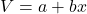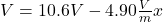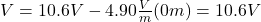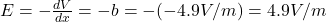## The potential in a region between x = 0 and x = 6.00 m is V = a + bx, where a = 10.6 V and b = -4.90 V/m. (a) Determine the potential at x =

Question

The potential in a region between x = 0 and x = 6.00 m is V = a + bx, where a = 10.6 V and b = -4.90 V/m. (a) Determine the potential at x = 0. 10.6 Correct: Your answer is correct. V Determine the potential at x = 3.00 m. V (b) Determine the magnitude and direction of the electric field at x = 0. magnitude 4.9 Correct: Your answer is correct. V/m direction +x Correct: Your answer is correct. Determine the magnitude and direction of the electric field at x = 3.00 m. magnitude V/m direction

in progress 0
5 months 2021-09-02T18:10:59+00:00 1 Answers 12 views 0

a) 10.6V

b) E = 4.9V/m, +x direction

c) E = 4.9V/m, +x direction

Explanation:

You have the following function:(1)

for the potential in a region between x=0 and x=6.00 m

a = 10.6 V

b = -4.90V/ma) for x=0 you obtain for V:b) The relation between the potential difference and the electric field can be written as:(2)

the direction is +x

c) The electric field is the same for any value of x between x=0 and x=6m.

Hence,

E = 4.9V/m, +x direction